##### Pre-Calculus For DummiesIf your pre-calculus teacher gives you any two nonconsecutive terms of a geometric sequence, you can find the general formula of the sequence as well as any specified term. For example, if the 5th term of a geometric sequence is 64 and the 10th term is 2, you can find the 15th term. Just follow these steps:

1. Determine the value of r.

You can use the geometric formula to create a system of two formulas to find r: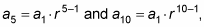or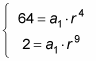You can use substitution to solve one equation for a1: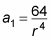Plug this expression in for a1 in the other equation: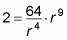Now simplify this equation: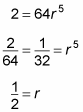2. Find the specific formula for the given sequence.

1. a.Plug r into one of the equations to find a1.

This step gives you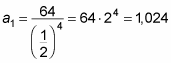2. b.Plug a1 and r into the formula.

Now that you know a1 and r, you can write the formula: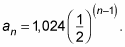3. Find the term you're looking for.

In this case, you want to find the 15th term (n = 15):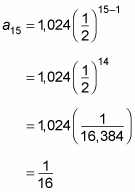Here's another example: The annual depreciation of a car's value is approximately 30 percent. Thus, every year, the car is actually worth 70 percent of its value from the year before. If a1 represents the value of a car when it was new and n represents the number of years that have passed,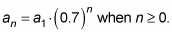Notice that this sequence starts at 0, which is okay as long as the information says that it starts at 0.